International Journal of Bioelectromagnetism Vol. 4, No. 2, pp. 83-84, 2002.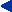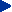# A GALERKIN BOUNDARY ELEMENT FORMULATION FOR CARDIAC ACTIVATION TIME IMAGING

G. Fischer1, B. Tilg1, R. Modre1, F. Hanser1,2, B. Messnarz1,2, P. Wach2
1University for Health Informatics and Technology Tyrol,
Innrain 98, A-6020 Innsbruck, AUSTRIA
e-mail: gerald.fischer@umit.at

2Institute of Biomedical Engineering, Graz University of Technology
Krenngasse 37, A-8010 Graz, AUSTRIA

Abstract: Cardiac activation time imaging provides information on the electrical activation sequence in the human heart by combining individual anatomical information (MR-imaging) and functional information (ECG-mapping). The inverse computation of the activation sequence for an individual beat is based on a multiple solution of the forward problem. Here, field computation techniques like the boundary element method are applied. Recent studies on cortical source localization showed that the Galerkin form of residual error weighting is superior to the commonly applied collocation form. We adopted the Galerkin method for our boundary element formulation. Numerical experiments on a spherical geometry showed that the accuracy improvement in the forward problem was almost one order of magnitude comparing the Galerkin vs. the collocation form in models of equal discretization.

INTRODUCTION

Detailed knowledge of the cardiac activation sequence of single beats in individual patients is essential for the interventional treatment of arrhythmias. Recently, the feasibility of noninvasive cardiac source imaging in humans was subject of validation studies for both the ventricles  and the atria . The inverse computation of an individual activation sequence is based on a multiple solution of the forward problem. An initially chosen activation pattern is iteratively modified in such a way that the computed forward solution fits the measured ECG data. Due to the ill-posed property of this problem regularization techniques have to be applied.

A high number of studies on this topic apply the boundary element method (BEM) for the forward calculation of the potential field -. Recent studies on cortical source localization (e.g. ) pointed out that the application of the Galerkin form of residual error weighting leads to a significant improvement in the forward solution with respect to the frequently used collocation form. The barrier, which has to be overcome applying the Galerkin approach, is an additional integration loop in the BEM software making the programmers task noticeable more complicated.

The hypothesis of this study is that an improvement in the forward solution should also improve the inverse solution. The Galerkin and collocation approach will be compared with an analytical reference solution on a concentric spheres model for a given activation pattern.

METHODS

Boundary element formulations are based on the bi-domain model and linear triangles are chosen as element type in both cases. Details on the governing equations can be found in . As a major advantage of the bidomain model the transmembrane potential V enters the source term linking known biophysical phenomena at cellular level to the BEM at a macroscopic scale. The computation of ECGs from a given activation sequence is performed in two steps:

Step a): A source pattern (transmembrane potential field) is obtained from the activation sequence at each time step. Here, a priori knowledge on the cellular action potential time course enters the calculation. When activated, the transmembrane potential V rises quickly from its resting value (-90mV) to plateau level (10mV). This is modeled by a step function.

Step b): The electric potentials j on the body surface are computed for each time step applying the BEM.

Note that it is possible to apply different forms of residual error weighting for each of these two steps. In this study we will use the term collocation for applying the collocation form to both steps. Analogously, the term Galerkin is used. No hybrid approaches are investigated.

Collocation

Step a): The a priori knowledge on the cardiac action potential time course is used in the element nodes only. Throughout each element the source pattern is obtained by linear interpolation of the transmembrane potential.

Step b): The governing integral equation is satisfied in the element nodes only. In all other points the evaluation of the integral equation will give a residual error as the interpolation functions for the electric potential are only an approximation for the true potentials .

Galerkin

Step a): The activation time in each point throughout the element is found by linear interpolation of the activation times in the element nodes. The a priori knowledge on the action potential time course can now be used in each point on the surface. We call the transmembrane potential, which is obtained from such a procedure in each point r and each time step t virtual transmembrane potential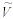(r, t) as it cannot be used directly for the solution of the forward problem. For a lead field matrix based forward solution the source pattern must be approximated by the according boundary element interpolation functions ni(r). The Galerkin approach can be applied for computing the transmembrane potentials in the nodes Vi(t) by: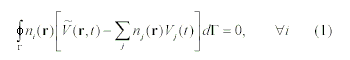Here, the surface of the heart is denoted by G. For summation we use the index j instead of i. The expression within the square brackets of (1) is the difference of the virtual transmembrane potential and its approximation. In other words this expression is equal to the error produced by the interpolation. The idea of the Galerkin approach is that the integral of these errors weighted by each interpolation function ni(r) should give zero (equally distributed positive and negative errors).

Equation (1) defines a linear system. Analogues to the application of the Galerkin method to finite elements the matrix on the left hand site is sparse, symmetric and positive definite. Note that in (1), only piecewise constant functions (virtual transmembrane potential) or linear functions (interpolation) appear, enabling a fast computation of all matrix coefficients.

Step b): The integral of the residual error of the integral equation weighted by each interpolation function ni(r ) should give zero (equally distributed positive and negative errors). Note that this requires an additional surface integral in the BEM computer code .

RESULTS

An analytic reference solution for a concentric spheres model with an axial-symmetric source pattern is presented in . The inner sphere represents the heart and the outer one the torso region. At t=0ms activation starts at the upper pole and spreads circular with constant velocity towards the lower pole which is reached at t=100ms. The reference solution in 62 regularly spaced electrodes on the chest is computed in time steps of 1ms. Three additional electrodes where applied for defining the reference lead by a Wilson terminal .

Three models of different level of discretization are investigated. The electric potentials in the 62 electrodes are computed for each time step applying the collocation and the Galerkin approach. The Wilson terminal is modeled by matrix deflation as suggested in . The relative error measure RE defined in  and  is used for quantitative comparison. In  RE is called relative difference measure. The results are listed in Table 1. The simulated ECG in one electrode is shown Fig. 1. Note the remarkable spikes in the signal computed by the collocation form. This can be explained at follows: for the collocation form the transmembrane potential in the nodes is rapidly 'switched' when the node is activated. The Galerkin form computes the nodes potentials from the transmembrane potential in the surrounding of each node. Here, a continuously propagating wave front leads to a continuously changing node potential. This smoothing removes the spikes at the body surface.

The increase in computation time due to an additional integration loop was relatively small. Most of the CPU time was consumed by the inversion of the BEM-matrices (which was equal for both formulations).

TABLE I
RELATIVE ERRORS RE

 model node spacing RE collocation RE Galerkin coarse 13 mm 5.68 % 1.18 % medium 9 mm 3.05 % 0.57 % fine 6 mm 1.86 % 0.26 %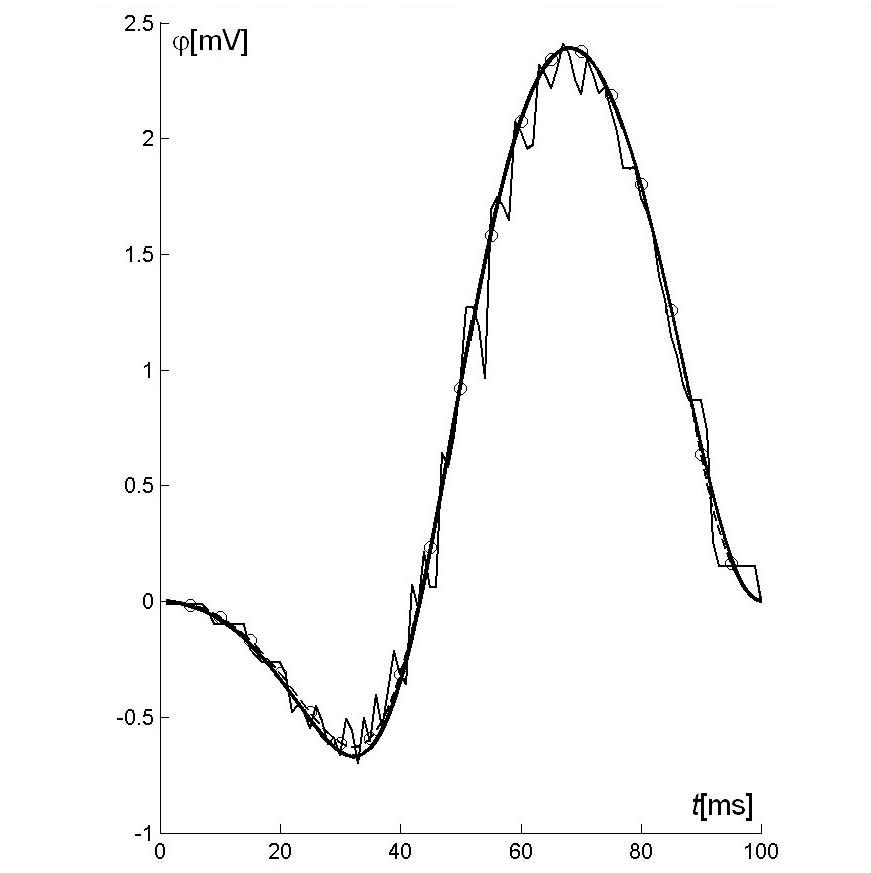Fig. 1: ECG computed in one electrode: analytic reference (bold line), collocation (narrow line) and Galerkin (broken line with circles). Results are shown for the coarse model.

DISCUSSION

The Galerkin form of residual error weighting leads to a significant improvement in the accuracy, which over compensates the increased computational cost. Results are presented for the forward problem. The influence of an improved forward solution on the inverse problem will be investigated in a future study.

Acknowledgments: This study was supported by the Austria Science Fund (FWF) under grant START Y144-INF.

##### REFERENCES

 R. Modre, B. Tilg B, G. Fischer, P. Wach. "Noninvasive myocardial activation time imaging: A novel inverse algorithm applied to clinical ECG mapping data," submitted to IEEE T. Biomed. Eng.

 B. Tilg B, G. Fischer, R. Modre, et. al.. "Model-based imaging of cardiac electrical excitation in humans," submitted to IEEE T. Med. Imag.

 G.J. Huiskamp, F. Greensite. "A new method for myocardial activation imaging," IEEE T. Biomed. Eng., vol. 44, pp. 433-446, 1997.

 J.C. Mosher, R.M. Leahy, P.S. Lewis."EEG and MEG: forward solutions for inverse methods," IEEE T. Biomed. Eng., vol. 46, pp. 245259, 1999.

 G.Fischer, B. Tilg, P. Wach, et al.. "Application of high-order boundary elements to the electrocardiographic inverse problem," Comput. Methods Programs Biomed., vol. 58, pp. 119-131, 1999.

 G.Fischer, B. Tilg, R. Modre, et. al.. "On Modeling the Wilson Terminal in the Boundary and Finite Element Method," IEEE T. Biomed. Eng., vol. 49, pp. 217-224, 2002.